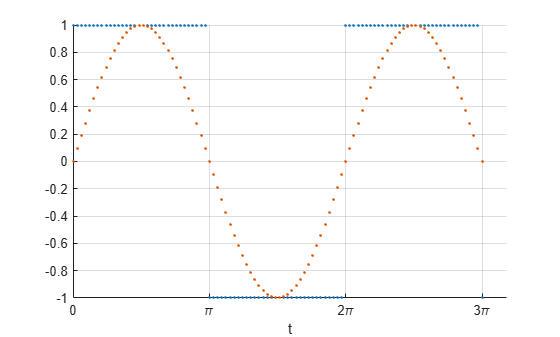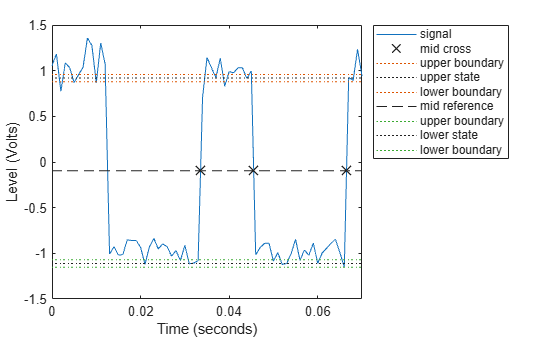square

Description

example

x = square(t) generates a square wave with period 2π for the elements of the time array t. square is similar to the sine function but creates a square wave with values of –1 and 1.

example

x = square(t,duty) generates a square wave with specified duty cycle duty. The duty cycle is the percent of the signal period in which the square wave is positive.

Examples

collapse all

Create a vector of 100 equally spaced numbers from $0$ to $3\pi$. Generate a square wave with a period of $2\pi$.

t = linspace(0,3*pi)';
x = square(t);

Plot the square wave and overlay a sine. Normalize the x-axis by $\pi$. The generated square wave has a value of $1$ for intervals [$\mathit{n}\pi ,\left(\mathit{n}+1\right)\pi$) with even $\mathit{n}$ and a value of $-1$ for intervals [$\mathit{n}\pi ,\left(\mathit{n}+1\right)\pi$) with odd $\mathit{n}$. The wave never has a value of $0$.

plot(t/pi,x,'.-',t/pi,sin(t))
xlabel('t / \pi')
grid onRepeat the calculation, but now evaluate square(2*t) at 121 equally spaced numbers between $-\pi$ and $2\pi$. Change the amplitude to $1.15$. Plot the wave and overlay a sine with the same parameters. This new wave is negative at $t=0$ and positive at the endpoints, $-\pi$ and $2\pi$.

t = linspace(-pi,2*pi,121);
x = 1.15*square(2*t);

plot(t/pi,x,'.-',t/pi,1.15*sin(2*t))
xlabel('t / \pi')
grid onGenerate a 30 Hz square wave sampled at 1 kHz for 70 ms. Specify a duty cycle of 37%. Add white Gaussian noise with a variance of 1/100.

t = 0:1/1e3:0.07;
y = square(2*pi*30*t,37)+randn(size(t))/10;

Compute the duty cycle of the wave. Plot the waveform and annotate the duty cycle.

dutycycle(y,t)ans = 0.3639

Input Arguments

collapse all

Time array, specified as a vector, matrix, or N-D array. square operates along the first array dimension of t with size greater than 1.

Data Types: single | double

Duty cycle, specified as a real scalar from 0 to 100.

Data Types: single | double

Output Arguments

collapse all

Square wave, returned as a vector, matrix, or N-D array.